• c++实验总结
千次阅读
2017-04-26 18:52:17

鉴于周五有c++模拟考试。。又是小崔出的全新的题目。。所以总结一下以前做过的实验和学过的重要的知识点或者思路；
Problem A: 学生比大小//没啥坑。。就是实验的测试用例不完全最开始的答案有bug重新改了之后应该没问题了

#include <iostream>
#include<string>
using namespace std;
class Student
{
public:
string name;
int age;
Student()
{
name="";
age=0;
}
int operator>(Student another)
{
if(age>another.age)
return 1;
else if(age==another.age)
{
int i=0;
while(i!=name.size()||i!=another.name.size())
{
if(name[i]>another.name[i])
return 1;
else if(name[i]==another.name[i])
i++;
else
return 0;
}
}
}
friend ostream& operator<<(ostream& os,Student& another);

friend istream& operator>>(istream& is,Student& another);

};
ostream& operator<<(ostream& os,Student& another)
{
cout<<another.name<<" "<<another.age<<endl;
return os;
}
istream& operator>>(istream& is,Student& another)
{
cin>>another.name>>another.age;
return is;
}
int main()
{
Student stu, max;
while(cin>>stu)
{
if (stu > max)
max = stu;
}
cout<<max<<endl;
return 0;
}

Problem B: 学生的排序：
最大的坑是老师main函数悄悄改掉了。。

#include <iostream>
#include<string>
#include<cstring>
#include<string.h>
#include <bits/stdc++.h>
using namespace std;
class Student
{
private:
string name;
int age;
public:
Student()
{
age=0;
name="";

}
int operator>(Student another)
{
if(age>another.age)
return 1;
else if(age==another.age)
{
int i=0;
while(i!=name.size()||i!=another.name.size())
{
if(name[i]>another.name[i])
return 1;
else if(name[i]==another.name[i])
i++;
else
return 0;
}
}
}
int operator<(Student another)
{
if(age<another.age||age==another.age)
return 1;
else
return 0;
}
friend istream &operator>>(istream& is,Student& stu);
friend ostream &operator<<(ostream& os,Student& stu);

};
class StudentGroup:public Student
{
public:
vector<Student> V;
{
V.push_back(tmp);
}
void srt()
{
vector<Student>::iterator iter1;
vector<Student>::iterator iter2;
Student tmp;
for(iter1=V.begin();iter1!=V.end();iter1++)
{
for(iter2=V.begin();iter2!=V.end();iter2++)
{
if(*iter1<*iter2)
{
tmp=*iter1;
*iter1=*iter2;
*iter2=tmp;
}
}
}
}
void show()
{
vector<Student>::iterator iter1;
for(iter1=V.begin();iter1!=V.end();iter1++)
{
cout<<*iter1;
}
}
};
istream &operator>>(istream& in,Student& s)
{
in>>s.name;
in>>s.age;
return in;
}
ostream &operator<<(ostream& os,Student& s)
{
cout<<s.name<<" "<<s.age<<endl;
return os;
}
int main()
{
Student stu, max;
StudentGroup stuG;
while(cin>>stu)
{
if (!(stu < max))
max = stu;
}
stuG.srt();
stuG.show();
cout<<"The max is "<<max<<endl;
return 0;
}


最后花了一个多小时就做了两道题我也很绝望啊。。

更多相关内容
• C++实验报告《面向对象程序课程设计》C++上机实验报告 为了方便，把代码放在Word里面了，每次上机实验的题目代码都在。 第一次： 对如下多项式编写类定义： + + +…+ 其中，n为多项式的次数。完成如下功能： （1）...
• 实验目的 掌握c++语言多态性的基本概念 掌握运算符重载函数声明和定义方法 运算符重载的函数格式： 函数类型 operator 运算符名称（形参表） { 对运算符的重载处理 } 运算符重载规则（1）除了少数的...

实验目的

掌握c++语言多态性的基本概念

掌握运算符重载函数声明和定义方法

运算符重载的函数格式：

函数类型 operator 运算符名称（形参表）

{

对运算符的重载处理

}

运算符重载规则
（1）除了少数的运算符不能重载(类属关系运算符“，”、作用域分辨符“：：”、三目运算符“？：”成员指针运算符“.*”)，大部分都可以重载。
（2）重载之后的运算符的优先级和结合性都不会改变。
（3）不能改变原运算符的操作对象个数，同事至少有一个操作对象是自定义类型。

实验内容

编写一个程序，实现两个复数的相乘

1.类外定义运算符重载函数

代码段：

#include<iostream>
using namespace std;
class Complex
{
public:
double real;
double imag;
Complex(double r = 0, double i = 0)
{
real = r; imag = i;
}
};
Complex operator * (Complex co1, Complex co2)
{
Complex temp;
temp.real = co1.real * co2.real - co1.imag * co2.imag;
temp.imag = co1.real * co2.imag + co1.imag * co2.real;
return temp;
}
int main()
{
float a, b, c, d;
cout << "请输入两个复数：" << endl;
cin >> a >> b >> c >> d;
Complex com1(a, b), com2(c, d), total1, total2;
total1 = operator*(com1, com2);
cout << "real1=" << total1.real << " " << "imag1=" << total1.imag << endl;
total2 = com1 * com2;
cout << "real2=" << total2.real << " " << "imag2=" << total2.imag << endl;
return 0;
}

运算结果：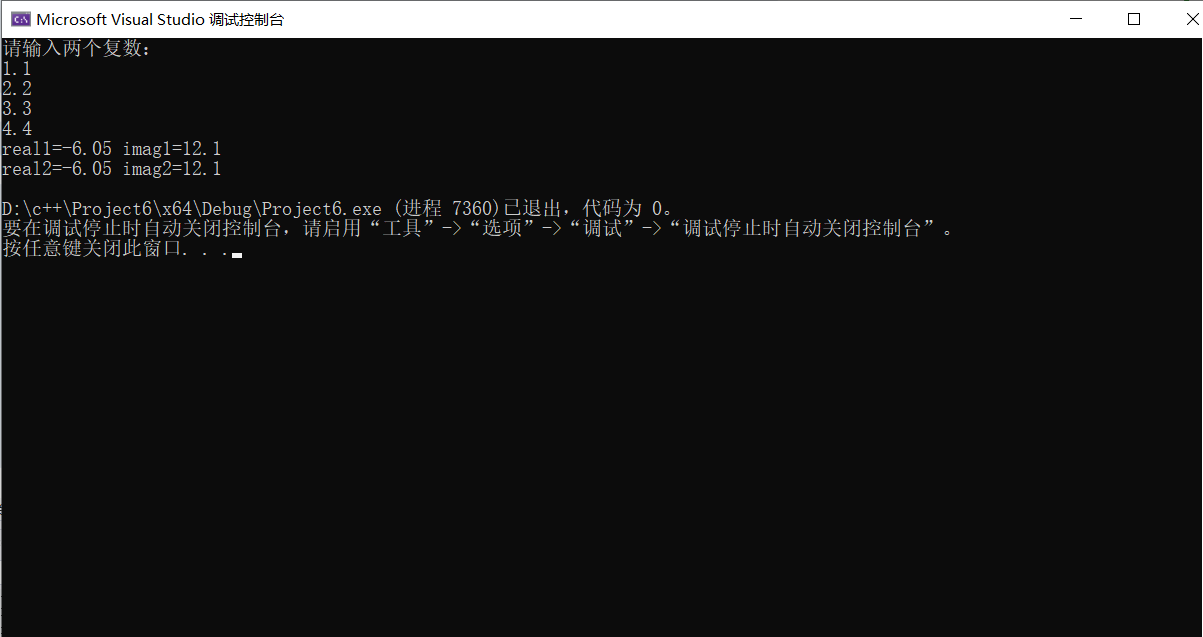2.友元运算符重载函数

代码段：

#include<iostream>
using namespace std;
class Complex
{
double real;
double imag;
public:
Complex(double r = 0, double i = 0)
{
real = r; imag = i;
}
void print();
friend Complex operator*(Complex co1, Complex co2);
};
Complex operator*(Complex co1, Complex co2)
{
Complex temp;
temp.real = co1.real * co2.real - co1.imag * co2.imag;
temp.imag = co1.real * co2.imag + co1.imag * co2.real;
return temp;
}
void Complex::print()
{
cout << "total real=" << real << " " << " total imag=" << imag << endl;
}
int main()
{
float a, b, c, d;
cout << "请输入两个复数：" << endl;
cin >> a >> b >> c >> d;
Complex com1(a, b), com2(c, d), total1;
total1 = com1 * com2;
total1.print();
return 0;
}

运算结果：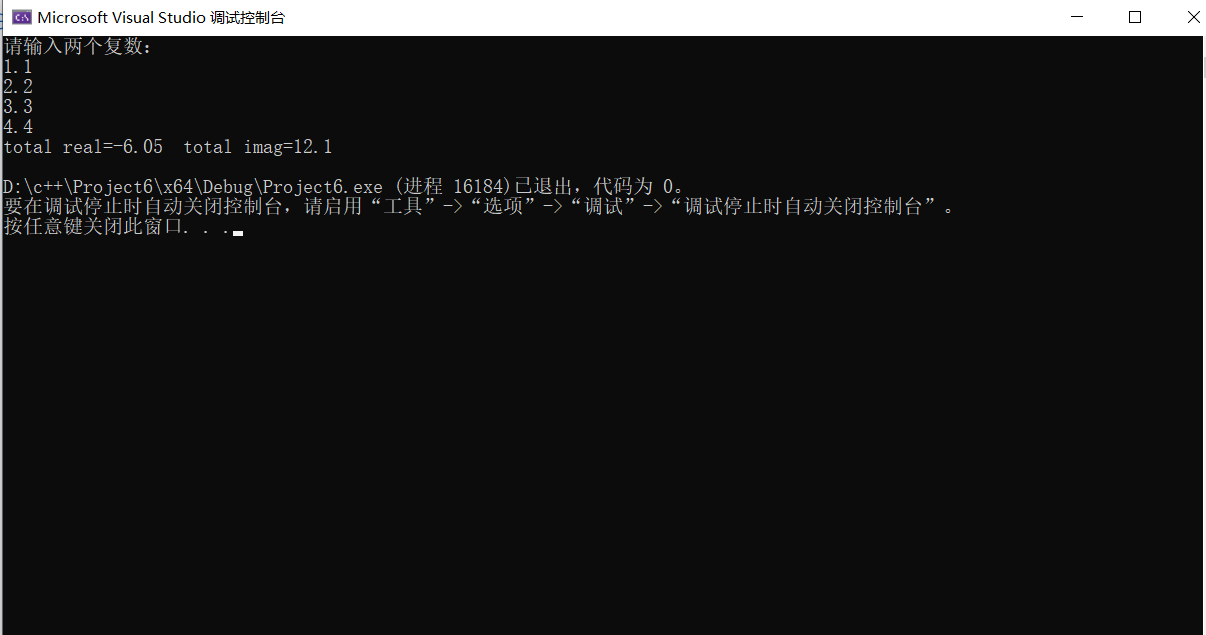在C++中，友元（friend）提供了在类外访问类中任意成员的方法（特权）

friend用于应对编程中一些比较特殊的情况，绝大多数情况下不需要使用，乱使用只会使C++变成C，甚至更糟。

友元函数虽然可以访问类对象的私有成员，但是不是成员函数，因此，在类的外部定义友元函数时，不需要"类名::".

3.成员运算符重载函数

代码段：

#include<iostream>
using namespace std;
class Complex
{
double real;
double imag;
public:
Complex(double r = 0.0, double i = 0.0);
void print();
Complex operator*(Complex c);
};
Complex::Complex(double r, double i)
{
real = r; imag = i;
}
Complex Complex :: operator*(Complex c)
{
Complex temp;
temp.real = real * c.real - imag * c.imag;
temp.imag = real * c.imag + imag * c.real;
return temp;
}
void Complex::print()
{
cout << "total real=" << real << "" << " total imag=" << imag << endl;
}
int main()
{
float a, b, c, d;
cout << "请输入两个复数：" << endl;
cin >> a >> b >> c >> d;
Complex com1(a, b), com2(c, d), total1;
total1 = com1 * com2;
total1.print();
return 0;
}

运算结果：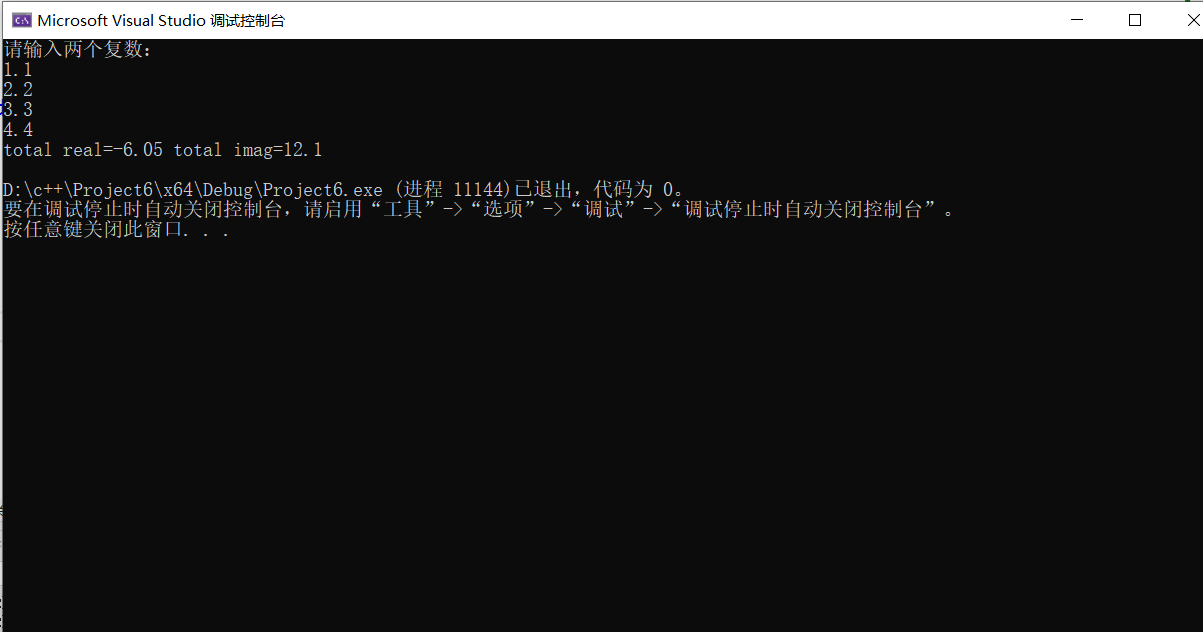对双目运算符而言，成员运算符重载函数的形参表中仅有一个参数，是运算符的右操作数。

另一个操作数（左操作数）是隐含的，是该类的当前对象，他是通过this指针隐含传递给函数。

注意事项：
1.除了类属关系运算符”.“、成员指针运算符”.*“、作用域运算符”::“、sizeof运算符和三目运算符”?:“以外，C++中的所有运算符都可以重载。
2.重载运算符限制在C++语言中已有的运算符范围内的允许重载的运算符之中，不能创建新的运算符。
3.运算符重载实质上是函数重载，因此编译程序对运算符重载的选择，遵循函数重载的选择原则。
4.重载之后的运算符不能改变运算符的优先级和结合性，也不能改变运算符操作数的个数及语法结构。
5.运算符重载不能改变该运算符用于内部类型对象的含义。它只能和用户自定义类型的对象一起使用，或者用于用户自定义类型的对象和内部类型的对象混合使用时。
6.运算符重载是针对新类型数据的实际需要对原有运算符进行的适当的改造，重载的功能应当与原有功能相类似，避免没有目的地使用重载运算符。

展开全文linq
• 实验目的 掌握派生类的声明方法函数与析构函数的执行顺序与构造规则

实验目的

掌握派生类的声明方法和派生类构造函数的定义方法

掌握不同方式下，构造函数与析构函数的执行顺序与构造规则

程序如下：

#include<iostream>
#include<string>
using namespace std;
class MyArray {
public:
MyArray(int length);
~MyArray();
void Input();
void Display(string);
protected:
int* alist;
int length;
};
MyArray::MyArray(int leng)
{
length = leng;
if (leng <= 0)
{
cout << "error length";
exit(1);
}
alist = new int[length];
if (alist == NULL)
{
cout << "assign failure";
exit(1);
}
cout << "MyArray类对象已创建!" << endl;
}
MyArray::~MyArray()
{
delete[] alist;
cout << "MyArray类对象已撤销!" << endl;
}
void MyArray::Display(string str)
{
int i;
int* p = alist;
cout << str << length << "个整数：";
for (i = 0; i < length; i++, p++)
cout << *p << "";
cout << endl;
}
void MyArray::Input()
{
cout << "请从键盘输入" << length << "个整数：";
int i;
int* p = alist;
for (i = 0; i < length; i++, p++)
cin >> *p;
}

class SortArray : public MyArray
{
public:
void Sort();
SortArray(int leng) :MyArray(leng)
{
cout << "SortArray类对象已创建!" << endl;
}
~SortArray();
};
SortArray:: ~SortArray()
{
cout << "SortArray类对象已撤销!" << endl;
}
void SortArray::Sort()
{
int i, j, temp;
for (i = 0; i < length - 1; i++)
for (j = 0; j < length - i - 1; j++)
{
if (alist[j] > alist[j + 1])
{
temp = alist[j];
alist[j] = alist[j+1];
alist[j + 1] = temp;
}
}
}
int main()
{
/*MyArray a(5);
a.Input();
a.Display("显示已经输入的");
return 0;*/
SortArray s(5);
s.Input();
s.Display("显示排序以前的");
s.Sort();
s.Display("显示排序以后的");
return 0;
}


实验内容：

声明一个storyarray继承类myarray，在该类中定义一个函数，具有将输入的整数从小到大进行排序的功能

class SortArray : public MyArray
{
public:
void Sort();
SortArray(int leng) :MyArray(leng)
{
cout << "SortArray类对象已创建!" << endl;
}
~SortArray();
};
SortArray:: ~SortArray()
{
cout << "SortArray类对象已撤销!" << endl;
}
void SortArray::Sort()
{
int i, j, temp;
for (i = 0; i < length - 1; i++)
for (j = 0; j < length - i - 1; j++)
{
if (alist[j] > alist[j + 1])
{
temp = alist[j];
alist[j] = alist[j+1];
alist[j + 1] = temp;
}
}
}

排序程序段:

int i, j, temp;
for (i = 0; i < length - 1; i++)
for (j = 0; j < length - i - 1; j++)
{
if (alist[j] > alist[j + 1])
{
temp = alist[j];
alist[j] = alist[j+1];
alist[j + 1] = temp;
}
}

运行结果：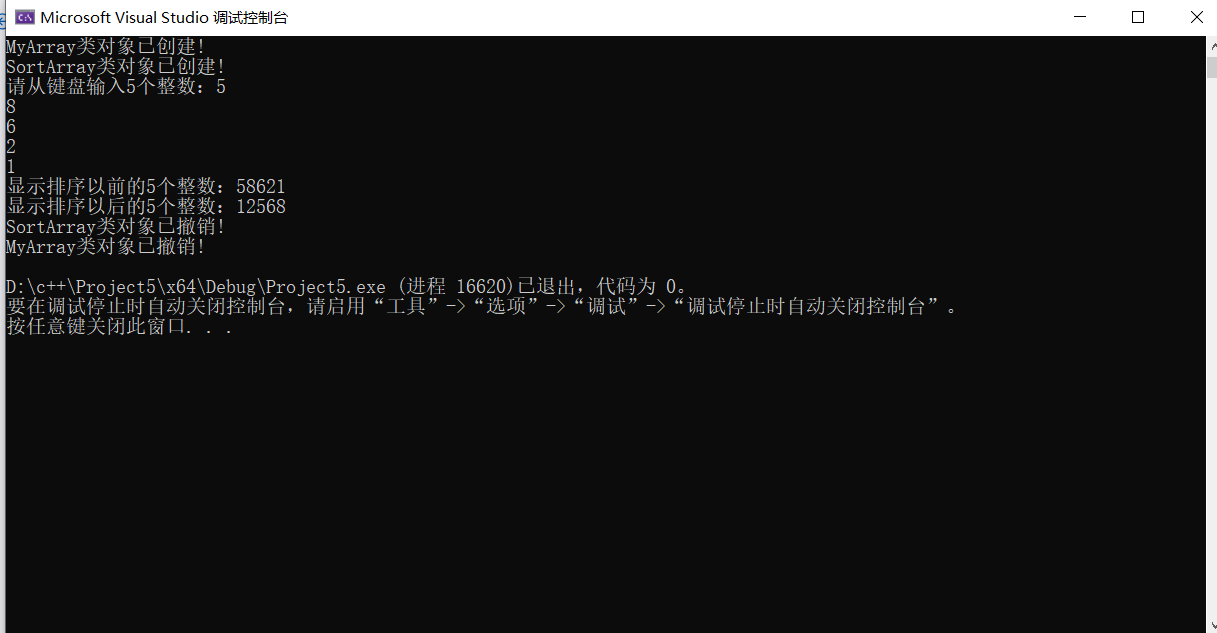构造函数的调用严格地按照先调用基类的构造函数，后调用派生类的构造函数的顺序执行。析构函数的调用顺序与构造函数的调用顺序正好相反，先调用派生类的析构函数，后调用基类的析构数。

派生类构造函数的一般格式为：
派生类名(参数总表):基类名(参数表) {
派生类新增数据成员的初始化语句
}
-----------------------------------------------------------------
含有子对象的派生类的构造函数：
派生类名(参数总表):基类名(参数表0),子对象名1(参数表1),...,子对象名n(参数表n)
{
派生类新增成员的初始化语句
}

在定义派生类对象时，构造函数的调用顺序如下：

调用基类的构造函数，对基类数据成员初始化。

调用子对象的构造函数，对子对象的数据成员初始化。

调用派生类的构造函数体，对派生类的数据成员初始化。

展开全文开发语言 后端
• 实验结果分析 该程序是利用C++输出两句话练习输出格式 实验C++简单程序设计 实验结果 实验结果分析 求一个图形的面积要先判断是哪一种图形用switch语句就可以解决再利用公式即可如输入1为圆形再输入边长3利用面积...
• ## c++实验报告

万次阅读 2017-03-12 17:36:16
第一个程序 一、问题及代码 /* *文件名称：Ex1-1.cpp ...*问题描述：我的第一个c++程序，熟悉程序的编辑、运行过程 *程序输出：y=3 *问题分析：可以使用if语句来解决这个问题 *算法设计：if语句 */ #incl

第一个程序

一、问题及代码

/*

*文件名称：Ex1-1.cpp

*作    者：李钊

*完成日期：2017312

*版 本 号：v1.0

*对任务及求解方法的描述部分：

*输入描述：输入x=8

*问题描述：我的第一个c++程序，熟悉程序的编辑、运行过程

*程序输出：y=3

*问题分析：可以使用if语句来解决这个问题

*算法设计：if语句

*/

#include<iostream>

#include<cmath>

using namespace std;

int main()

{

int x,y;

cout<<"请输入x=";cin>>x;

if (x<2)

{

y=x;

}

else if((x>=2)&&(x<6))

{

y=x*x+1;

}

else if((x>=6)&&(x<10))

{

y=sqrt(x+1);

}

else

{

y=1/(x+1);

}

cout<<"y="<<y<<endl;

}

二、运行结果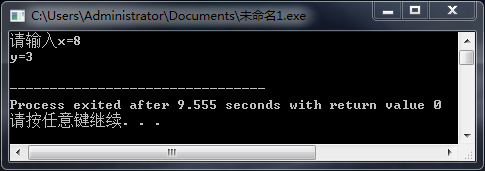三、心得体会：

第一个c++程序，中间有些小障碍，成功克服。

c++，我会好好学的！

四、知识点总结：

一个程序至少要有一个函数，main函数常称为主函数；函数中所有语句都包括在一对花括号中。

第二个程序

一、问题及代码

/*

*文件名称：Ex1-1.cpp

*作    者：李钊

*完成日期：2017312

*版 本 号：v1.0

*对任务及求解方法的描述部分：

*输入描述：无输入

*问题描述：我的第一个c++程序，熟悉程序的编辑、运行过程

*程序输出：鸡翁的只数，鸡母的只数，鸡雏的只数

*问题分析：可以使用for语句来解决这个问题

*算法设计：for语句

*/

#include<iostream>
using namespace std;
int main()
{
int x,y,z;
for(x=0;x<=20;x++)
{
for(y=0;y<=33;y++)
{
for(z=0;z<=100;z++)
{
if((5*x+3*y+z/3==100)&&(x+y+z==100))
{
cout<<"鸡翁只数"<<x<<"鸡母只数"<<y<<"鸡雏只数"<<z<<endl;
}
}
}
}
}

二、运行结果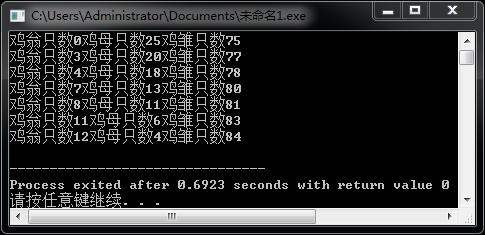三、心得体会：

第一个c++程序，中间有点小障碍，成功克服。

c++，我会好好学的！

四、知识点总结：

一个程序至少要有一个函数，main函数常称为主函数；函数中所有语句都包括在一对花括号中。

展开全文• ## c++实验心得

千次阅读 2020-05-20 15:23:38
拷贝文件到另一文件中单位应为char string过不去 其他未试过 机理未知 拷贝中读取默认忽略空白符 若需要不忽略 应加>>std::noskipws 如： infile>>std::noskipws>>m
• 程序运行结果： 使用默认值时： 用户自己设置输入坐标值时（以8为例）： 实验总结 ： 本次实验掌握了构造函数的实现方法，掌握了类中的三种限定符 public，private，protected，private、protected类的成员不能从类...开发语言 后端
• 实验目的： 掌握文件流的概念 掌握文件的打开、关闭以及各种函数的使用方法 掌握文件的读写方法 实验内容 在实验2－继承与派生的基础上添加以下功能： 把student类和teacher类对象的信息保存到文本文件中 从文本文件...
• c++基础知识总结，适合初学者 1 static（静态）变量有什么作用 3个体明显的作用：1）在函数体内，静态变量具有“记忆”功能，即一个被声明为静态变量在一个函数被调用的过程中其值维持不变2）在模块内，它的作用域...
• 实验报告模板c++上机可以方便大家认真总结每一次的上机情况
• C++实训总结报告-最新范文 为期一个星期的c++实训已经基本结束但是给了我很大的影响通过这次实训使我明白C++语言这门课程光仅仅是听课是远远不够的上机训练也不容忽视 通过上机训练才能够明白自己知识的不足才能够...
• 组合逻辑电路（半加器全加器及逻辑运算） 一、实验目的 1．掌握组合逻辑电路功能调试的基本方法。 2．验证半加器和全加器的逻辑功能。 3．复习二进制数的运算规律。二、实验设备和器件1. 在线实验教学智能考核系统、...
• 实验一 类与对象 实验目的 掌握类的声明和使用 掌握类的声明和对象的声明 复习具有不同访问属性的成员的访问方式 观察构造函数和析构函数的执行过程 学习类的组合使用方法 使用VC++的debug调试功能观察程序流程跟踪...
• 实验内容： 1、将任意十进制数转换为...文件包含源代码和实验报告，环境为vs2019，实验报告中含有实验目的，实验分析，实验源代码，实验运行结果，注释较多，对基础小白较友好。可用于提交数据结构顺序栈的作业和学习。
• 1、熟悉Visual C++开发环境； 2、掌握MFC编程基础； 3、掌握CDC图形库； 4、利用CDC图形库函数绘制简单图形。 二、实验性质 验证性 三、实验要求 1、认真阅读本次实验的目的，清楚本次实验要求和实验内容； 2、能够...
• 实验四 输入输出流 实验课程名面向对象程序设计 C++ 专业班级 学号 姓名 实验时间 实验地点 指导教师 一实验 目的和要求 (1) 理解类和对象的概念掌握声明类和定义对象的 方法 (2) 掌握构造函数和析构函数的实现方法 ...
• 实验十 常用控件 1 实验目的和要求 1创建一个默认的对话框应用程序 Ex_Ctrls 如图 2.1 所示 2 设计一个如图 2.2 所示的课程信息对话框 图 2.1 Ex_Ctrls 对话框 图 2.2 课程信息对话框 3 实现功能单击图 2.1 中的...
• C++二叉树的创建与遍历实验报告 作者: 日期 ? 二叉树的创建与遍历 一 实验目的 1.学会实现二叉树结点结构和对二叉树的基本操作 2.掌握对二叉树每种操作的具体实现学会利用递归和非递归方法编写对二叉树这种递归数据...
• C++: 16.03.04实验总结标签： C++ 实验课by 小威威 1.缺省构造函数缺省构造函数主要有两种类型：(1)无形参；(2)有形参。class Student { public: Student(); // 无形参 Student(int a1, int b1 ＝ 0, int c1 ＝...缺省构造函数 snprintf sstream
• ## c++实验报告--系列

千次阅读 2019-05-04 22:01:50
实验名称 运算符的重载 指导教师 实验类型 （软件、硬件） 软件 实验学时 2学时 实验时间 2019．4．...
• c++ 实验报告参考 代码问题 关于c++中虚函数，数组，指针问题
• 面向对象程序设计 C++语言课程设计 设计说明书 图书管理系统的设计与实现 起止日期 年 月 日 至 年 月 日 学 生 姓 名 班 级 学 号 成 绩 指 导 教 师 ( 签 字 ) 计算机与通信学院 年 月 日 图书管理系统 一 ....
• C++图的深度遍历及广度遍历的代码 及最小生成树的操作
• C++的学习心得 注意因为我们在实验室大多是以项目为基础来学习C++这样可能导致我们不能形成一个知识体系所以建议大一同学脚踏实地的从头学习C++即使时间不充裕的大二大三同学在学习时也要做好笔记以便下次遇到同样的...
• Visual C++ 三、实验内容： (写出主要的内容) 1． 定义Point类，有坐标x，y两个成员变量；对Point类重载＋、－、++（左）、++（右）四种运算符，实现对坐标值的运行与改变。 #include<iostream>
• 实验目的： ①.进一步加深对类和对象的理解; ②.掌握集中对象传递的使用方法; ③.掌握静态成员的概念和使用; 实验共分成三部分，分别为part a,part b,part c; 实验内容： part a: 了解三种不同对象传递...开发语言...

# c++实验总结c++ 订阅